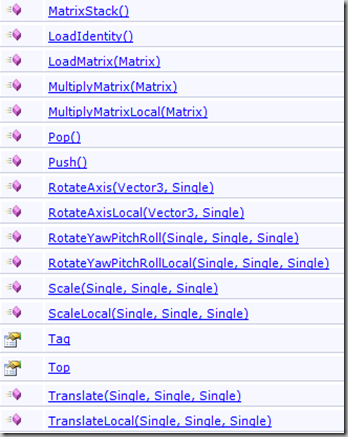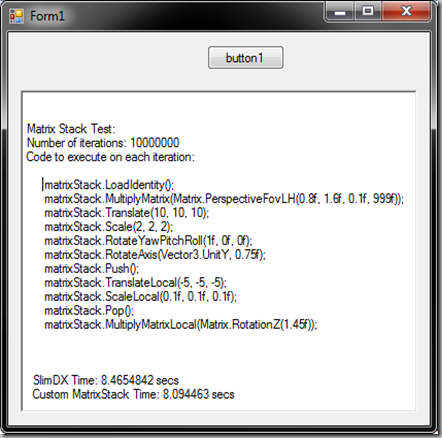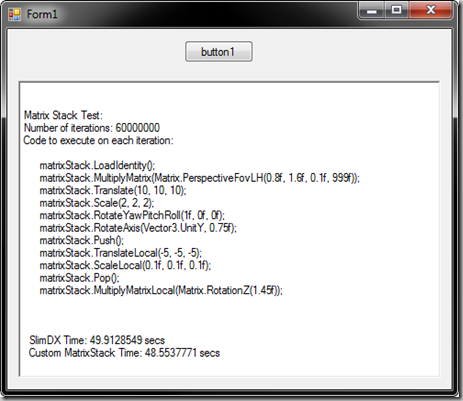## Friday, 23 March 2012

### Developing a MatrixStack in pure managed C# code (ready for XNA)

Some time ago, we already talked about the possibility of creating your own Math library directly in C#, with no native code. If you take enough care, it can be as fast as performing interop with a native one.Today, we are showing an additional example on this matter, and we are going to develop our own fast MatrixStack class, all in safe C# code, with no COM interop.

#### Why?

I never understood well why the MatrixStack class remains to be an iDisposable COM object. Don´t know what kind of optimizations it has internally that justify having disposable resources, but it’s annoying to have the iDisposable overhead with no need for it.
Besides that, MatrixStacks are used in most cases as simple matrix helpers, to traverse object hierarchies. So, replacing the API MatrixStack with your own one should be a piece of cake, and will definitely help you if trying to port your code to some other platform.
Last, but not least, XNA does not have a MatrixStack class. So this C# implementation fits perfectly on it for all that want to use it.
I this example, I will be comparing my own class with the SlimDX MatrixStack, which is nothing more than a wrapper over the D3DX Matrix Stack.

#### The interface

In order to make the SlimDX stack replacement painless, I will keep the exact same interface in my class (except the COM-related stuff, which is no longer necessary). So, it will have to be something like this:#### How it works

A MatrixStack, basically supplies a mechanism to enable matrices to be pushed onto and popped off of a matrix stack. Implementing a matrix stack is an efficient way to track matrices while traversing a transform hierarchy.
So, we can clear the stack to the Identity or to any other matrix, we can operate with the top of the stack, and we can add (push) or remove (pop) nodes (or levels, if you want) to the stack.
Example: for a robot arm hierarchy, we would go like this:1.- Initialize the stack, and load the matrix of the first node in the hierarchy (the upper arm, for example). Now you can use the Top matrix to draw the upper arm.
2.- Create another level on the stack (Push) for the lower arm, and multiply the lower arm matrix. Use the Top matrix to draw the lower arm.
3.- Create another level on the stack (Push) for the hand, and multiply the hand matrix. Use the Top matrix to draw the hand.
The stack itself does nothing you cannot do with regular Matrix multiplications, except that it keeps track of the previous levels you have been creating. So you can go back to the upper node whenever you want. After the previous operations, for instance, if we perform a Pop, we would remove the top node of the stack, and go back to the previous. This way, the new Top node would represent the lower arm matrix, instead of the hand matrix.

#### The code

Here is my implementation of the MatrixStack. Please keep in mind that it has not been intensively tested, and might contain errors. Use it at your own risk:
public class MatrixStack
{
/// <summary>
/// Retrieves the Top node matrix of the stack
/// </summary>
public Matrix Top = Matrix.Identity;
public object Tag = null;
private List<Matrix> mStack = new List<Matrix>();

/// <summary>
///
/// </summary>
public MatrixStack()
{
}
/// <summary>
/// Clears the stack and loads the Identity Matrix in the top of the stack
/// </summary>
{
mStack.Clear();
Top = Matrix.Identity;
}
/// <summary>
/// Clears the Stack, and loads the matrix in the top of the stack
/// </summary>
/// <param name="pMat"></param>
public void LoadMatrix(Matrix pMat)
{
mStack.Clear();
Top = pMat;
}
/// <summary>
/// Adds a new level to the stack, cloning the current TOP matrix of the stack
/// </summary>
public void Push()
{
}
/// <summary>
/// Removes the current TOP matrix of the stacks, returning back to the previous one
/// </summary>
public void Pop()
{
if (mStack.Count > 0)
{
Top = mStack[mStack.Count - 1];
mStack.RemoveAt(mStack.Count - 1);
}
}
/// <summary>
/// This method right-multiplies the given matrix to the current matrix (transformation is about the current world origin).
/// This method does not add an item to the stack, it replaces the current matrix with the product of the current matrix and the given matrix.
/// </summary>
/// <param name="pMat"></param>
public void MultiplyMatrix(Matrix pMat)
{
Matrix.Multiply(ref Top, ref pMat, out Top);
}
/// <summary>
/// This method left-multiplies the given matrix to the current matrix (transformation is about the local origin of the object).
/// This method does not add an item to the stack, it replaces the current matrix with the product of the given matrix and the current matrix.
/// </summary>
/// <param name="pMat"></param>
public void MultiplyMatrixLocal(Matrix pMat)
{
Matrix.Multiply(ref pMat, ref Top, out Top);
}
/// <summary>
/// Rotates (relative to world coordinate space) around an arbitrary axis.
/// </summary>
public void RotateAxis(Vector3 pAxis, float pAngle)
{
Matrix tmp;
Matrix.RotationAxisAngle(ref pAxis, pAngle, out tmp);
Matrix.Multiply(ref Top, ref tmp, out Top);
}
/// <summary>
/// Rotates (relative to world coordinate space) around an arbitrary axis.
/// </summary>
public void RotateAxisLocal(Vector3 pAxis, float pAngle)
{
Matrix tmp;
Matrix.RotationAxisAngle(ref pAxis, pAngle, out tmp);
Matrix.Multiply(ref tmp, ref Top, out Top);
}
/// <summary>
/// Rotates (relative to world coordinate space) the specified Euler Angles
/// </summary>
public void RotateYawPitchRoll(float pYaw, float pPitch, float pRoll)
{
Matrix tmp;
Matrix.CreateFromYawPitchRoll(pYaw, pPitch, pRoll, out tmp);
Matrix.Multiply(ref Top, ref tmp, out Top);
}
/// <summary>
/// Rotates (relative to world coordinate space) the specified Euler Angles
/// </summary>
public void RotateYawPitchRollLocal(float pYaw, float pPitch, float pRoll)
{
Matrix tmp;
Matrix.CreateFromYawPitchRoll(pYaw, pPitch, pRoll, out tmp);
Matrix.Multiply(ref tmp, ref Top, out Top);
}
/// <summary>
/// Scale the current matrix about the world coordinate origin
/// </summary>
public void Scale(float pX, float pY, float pZ)
{
Matrix tmp;
Matrix.CreateScale(pX, pY, pZ, out tmp);
Matrix.Multiply(ref Top, ref tmp, out Top);
}
/// <summary>
/// Scale the current matrix about the world coordinate origin
/// </summary>
public void ScaleLocal(float pX, float pY, float pZ)
{
Matrix tmp;
Matrix.CreateScale(pX, pY, pZ, out tmp);
Matrix.Multiply(ref tmp, ref Top, out Top);
}
/// <summary>
/// Determines the product of the current matrix and the computed translation matrix determined by the given factors (x, y, and z).
/// </summary>
public void Translate(float pX, float pY, float pZ)
{
Matrix tmp;
Matrix.CreateTranslation(pX, pY, pZ, out tmp);
Matrix.Multiply(ref Top, ref tmp, out Top);
}
/// <summary>
/// Determines the product of the current matrix and the computed translation matrix determined by the given factors (x, y, and z).
/// </summary>
public void TranslateLocal(float pX, float pY, float pZ)
{
Matrix tmp;
Matrix.CreateTranslation(pX, pY, pZ, out tmp);
Matrix.Multiply(ref tmp, ref Top, out Top);
}
}

#### It has to be fast

When you start coding your own MatrixStack, you will soon realize that .Net includes a Generic Collection called Stack. You can use it, although I didn’t. Why?
Because I have separated the management of the Top Matrix of the stack to a member variable, and for the rest I just preferred to use a simple list to keep track of the previous nodes.
The Top Matrix is stored as a member variable to be able to pass it By Reference to the Matrix Multiplication methods. The speed increase avoiding to pass a whole matrix by value is significant. In the example below, it was around a 40% faster.

#### Test 1 – Reliability

I just made several random operations with the matrix stack, trying to test some of its features by comparing the end Top Matrix, both with a SlimDX MatrixStack and my own. The test operations are:
matrixStack.MultiplyMatrix(Matrix.PerspectiveFovLH(0.8f, 1.6f, 0.1f, 999f));
matrixStack.Translate(10, 10, 10);
matrixStack.Scale(2, 2, 2);
matrixStack.RotateYawPitchRoll(1f, 0f, 0f);
matrixStack.RotateAxis(Vector3.UnitY, 0.75f);
matrixStack.Push();
matrixStack.TranslateLocal(-5, -5, -5);
matrixStack.ScaleLocal(0.1f, 0.1f, 0.1f);
matrixStack.Pop();
matrixStack.MultiplyMatrixLocal(Matrix.RotationZ(1.45f));
The resulting top matrix is:
SlimDX MatrixStack:
1. [M11:-0.06350367 M12:4.695973 M13:-0.3505643 M14:0]
2. [M21:0.5231493 M22:0.5700315 M23:2.887983 M24:0]
3. [M31:18.08297 M32:20 M33:-23.60117 M34:1]
4. [M41:-0.1968169 M42:0 M43:0.03565279 M44:0]
MyMatrixStack:
1. {M11:-0.06350368 M12:4.695973 M13:-0.3505643 M14:0}
2. {M21:0.5231493 M22:0.5700315 M23:2.887982 M24:0}
3. {M31:18.08297 M32:20 M33:-23.60117 M34:1}
4. {M41:-0.1968169 M42:0 M43:0.0356528 M44:0}
As you can see, the result is exactly the same.

#### Test 2 - Speed

Speed is important, so I decided to run the above mentioned operation 10 million times, to se how long it takes to complete both using SlimDX and my own code.
Obviously, if we run in Debug mode (disabling optimizations), there will be a huge performance difference, as the SlimDX dll is already compiled with optimizations. But what happens if we turn all optimizations on when compiling our code?
Here is the result of a small test application:As you can see, the .Net Framework alone is faster than SlimDX, thanks to its optimizations and to the absence of the interop layer.
What happens if we increase the number of iterations to 60 million? The difference is obviously bigger (1.36 seconds faster):Note: This test has been done on an intel i7 CPU at 3.8 Ghz, running on Windows 7 x64 with .Net Framework 4.0.
Note2: SlimDX MatrixStack uses its own Matrix class and operations. My implementation uses my own Matrix implementation, also written in pure C# code.
Conclusion: .Net Rocks. A purely native C++ code would be even faster of course, but if you put in the equation the huge amount of benefits .Net will give you, I really think it’s worth it. Don’t you think?
Cheers !